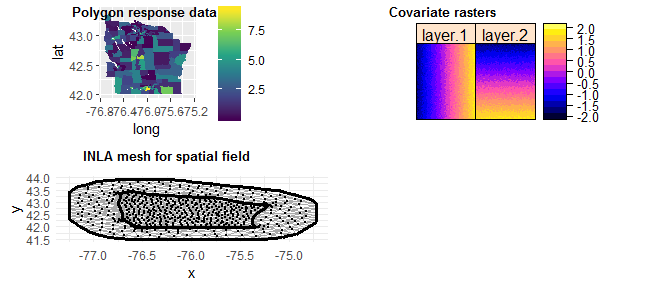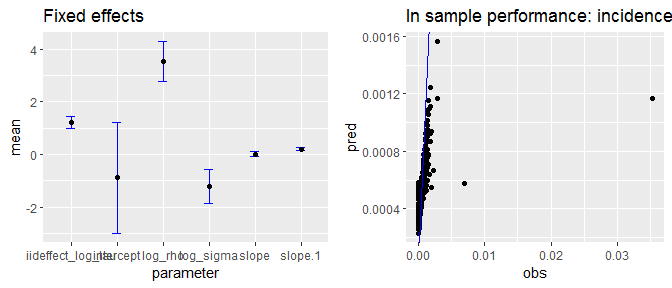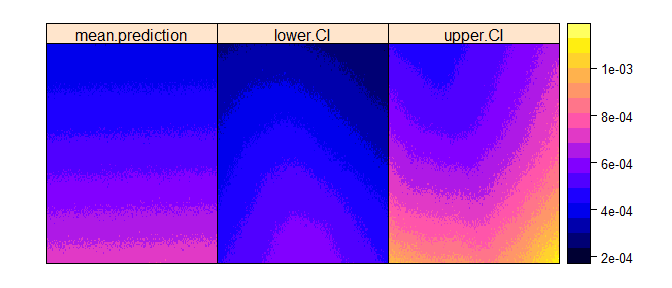# A short introduction to the disaggregation package

#### 2020-02-18

The disaggregation package contains functions to run Bayesian disaggregation models. Aggregated response data over large heterongenous regions can be used alongside fine-scale covariate information to predict fine-scale response across the region. The github page for this package can be found here.

Install disggregation using:

devtools::install_github("aknandi/disaggregation")

The key functions are prepare_data, fit_model and predict. The prepare_data function takes the aggregated data and covariate data to be used in the model and produces an object to be use by fit_model. This functions runs the disaggregation model and the out can be passed to predict to produce fine-scale predicted maps of the response variable.

To use the disaggregation prepare_data fuction, you must have the aggregated data as a SpatialPolygonDataFrame object and a RasterStack of the covariate data to be used in the model.

## Example

We will demonstrate an example of the disaggregation package using areal data of leukemia incidence in New York, using data from the package SpatialEpi.

library(SpatialEpi, quietly = TRUE)
library(dplyr, quietly = TRUE)
library(sp, quietly = TRUE)
library(raster, quietly = TRUE)
library(disaggregation, quietly = TRUE)

map <- NYleukemia$spatial.polygon df <- NYleukemia$data

polygon_data <- SpatialPolygonsDataFrame(map, df)
polygon_data
#> class       : SpatialPolygonsDataFrame
#> features    : 281
#> extent      : -76.73743, -75.2395, 41.9978, 43.41827  (xmin, xmax, ymin, ymax)
#> crs         : +proj=longlat
#> variables   : 3
#> names       : censustract.FIPS,   cases, population
#> min values  :      36007000100, 0.00014,          9
#> max values  :      36109992300, 9.28601,      13015

Now we simulate two covariate rasters for the area of interest and make a RasterStack. They are simulated at the resolution of approximately 1km2.

extent_in_km <- 111*(polygon_data@bbox[, 2] - polygon_data@bbox[, 1])
n_pixels_x <- floor(extent_in_km[])
n_pixels_y <- floor(extent_in_km[])
r <- raster::raster(ncol = n_pixels_x, nrow = n_pixels_y)
r <- raster::setExtent(r, raster::extent(polygon_data))
r[] <- sapply(1:raster::ncell(r), function(x) rnorm(1, ifelse(x %% n_pixels_x != 0, x %% n_pixels_x, n_pixels_x), 3))
r2 <- raster::raster(ncol = n_pixels_x, nrow = n_pixels_y)
r2 <- raster::setExtent(r2, raster::extent(polygon_data))
r2[] <- sapply(1:raster::ncell(r), function(x) rnorm(1, ceiling(x/n_pixels_y), 3))
cov_stack <- raster::stack(r, r2)
cov_stack <- raster::scale(cov_stack)

We also create a population raster. This is to allow the model to correctly aggregated the pixel values to the polygon level. For this simple example we assume that the population within each polygon is uniformly distributed.

extracted <- raster::extract(r, polygon_data)
n_cells <- sapply(extracted, length)
polygon_data@data$pop_per_cell <- polygon_data@data$population/n_cells
pop_raster <- rasterize(polygon_data, cov_stack, field = 'pop_per_cell')

To correct small inconsistencies in the polygon geometry, we run the line below

polygon_data <- rgeos::gBuffer(polygon_data, byid = TRUE, width = 0)
#> Warning in rgeos::gBuffer(polygon_data, byid = TRUE, width = 0): Spatial object
#> is not projected; GEOS expects planar coordinates

Now we have setup the data we can use the prepare_data function to create the objects needed to run the disaggregation model. The name of the response variable and id variable in the SpatialPolygonsDataFrame should be specified.

The user can also control the parameters of the mesh that is used to create the spatial field. The mesh is created by finding a tight boundary around the polygon data, and creating a fine mesh within the boundary and a coarser mesh outside. This speeds up computation time by only having a very fine mesh within the area of interest and having a small region outside with a coarser mesh to avoid edge effects. The mesh parameters: concave, convex and resolution refer to the parameters used to create the mesh boundary using the inla.noncovex.hull function, while the mesh parameters max.edge, cut and offset refer to the parameters used to create the mesh using the inla.mesh.2d function.

data_for_model <- prepare_data(polygon_data,
cov_stack,
pop_raster,
response_var = 'cases',
id_var = 'censustract.FIPS',
mesh.args = list(cut = 0.01,
offset = c(0.1, 0.5),
max.edge = c(0.1, 0.2),
resolution = 250),
na.action = TRUE,
ncores = 1)
#> Warning: 'cBind' is deprecated.
#>  Since R version 3.2.0, base's cbind() should work fine with S4 objects
plot(data_for_model)Now have our data object we are ready to run the model. Here we can specify the likelihood function as gaussian, binomial or poisson, and we can specify the link function as logit, log or identity. The disaggregation model makes predictions at the pixel level:

$$link(pred_i) = \beta_0 + \beta X + GP(s_i) + u_i$$

where $$X$$ are the covariates, $$GP$$ is the gaussian random field and $$u_i$$ is the iid random effect. The pixel predictions are then aggregated to the polygon level using the weighted sum (via the aggregation raster, $$agg_i$$):

$$cases_j = \sum_{i \epsilon j} pred_i \times agg_i$$

$$rate_j = \frac{\sum_{i \epsilon j} pred_i \times agg_i}{\sum_{i \epsilon j} agg_i}$$

The different likelihood correspond to slightly different models ($$y_j$$ is the repsonse count data):

Gaussian ($$\sigma_j$$ is the dispersion of the polygon data),

$$dnorm(y_j/\sum agg_i, rate_j, \sigma_j)$$

Here $$\sigma_j = \sigma \sqrt{\sum agg_i^2} / \sum agg_i$$, where $$\sigma$$ is the dispersion of the pixel data, a parameter learnt by the model.

Binomial (For a survey in polygon j, $$y_j$$ is the number positive and $$N_j$$ is the number tested)

$$dbinom(y_j, N_j, rate_j)$$

Poisson (predicts incidence count)

$$dpois(y_j, cases_j)$$

The user can also specify the priors for the regression parameters. For the field, the user specifies the pc priors for the range, $$\rho_{min}$$ and $$\rho_{prob}$$, where $$P(\rho < \rho_{min}) = \rho_{prob}$$, and the variation, $$\sigma_{min}$$ and $$\sigma_{prob}$$, where $$P(\sigma > \sigma_{min}) = \sigma_{prob}$$, in the field. For the iid effect, the user also specifies pc priors.

By default the model contains a spatial field and a polygon iid effect. These can be turned off in the fit_model function, using field = FALSE or iid = FALSE.

model_result <- disag_model(data_for_model,
iterations = 1000,
family = 'poisson',
link = 'log',
priors = list(priormean_intercept = 0,
priorsd_intercept = 2,
priormean_slope = 0.0,
priorsd_slope = 0.4,
prior_rho_min = 3,
prior_rho_prob = 0.01,
prior_sigma_max = 1,
prior_sigma_prob = 0.01,
prior_iideffect_sd_max = 0.05,
prior_iideffect_sd_prob = 0.01))
#> Fitting model. This may be slow.
#> Fitting model. This may be slow.
#> Warning in disag_model(data_for_model, iterations = 1000, family = "poisson", :
#> The model did not converge. Try increasing the number of iterations
plot(model_result)Now we have the results from the model of the fitted parameters, we can predict Leukemia incidence rate at fine-scale (the scale of the covariate data) across New York. The predict function takes the model result and predicts both the mean raster surface and predicts and summarises N parameter draws, where N is set by the user (default 100). The uncertainty is summarirised via the confidence interval set by the user (default 95% CI).

preds <- predict(model_result,
N = 100,
CI = 0.95)

plot(preds)